# Analyzing Motion in Connected Rigid Bodies (Part - 2) Civil Engineering (CE) Notes | EduRev

## Civil Engineering (CE) : Analyzing Motion in Connected Rigid Bodies (Part - 2) Civil Engineering (CE) Notes | EduRev

The document Analyzing Motion in Connected Rigid Bodies (Part - 2) Civil Engineering (CE) Notes | EduRev is a part of the Civil Engineering (CE) Course Introduction to Dynamics and Vibrations- Notes, Videos, MCQs.
All you need of Civil Engineering (CE) at this link: Civil Engineering (CE)

6.3.1 Summary of constraint equations at joints and contacts

As the examples in the preceding section show, the keys to analyzing motion in a system of connected rigid bodies are: (1) the formulas for relative velocity and acceleration of two points in a rigid body, and (2) constraints that relate the velocities and accelerations on two bodies at points where they touch.

There are three common types of connection between rigid bodies:

1. A pin joint: the two connected members must have the same velocity and acceleration at the connected point

v- vA    aB - aA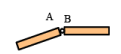2. A slider joint: the two connected members must have the same velocity and acceleration normal to the slider

vB • n = vA• n    aB • n = aA • n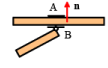3. Contact between two objects without relative slip (sliding) at the contact (friction forces must act to prevent the slip, in general):  The velocities of the touching objects must be equal at the contact point.   The tangential components of acceleration must also be equal (the normal components of acceleration differ)

vB = vA    aB • t = aA • t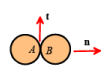6.3.2 The Rolling Wheel

Wheels are everywhere.   They can be analyzed using the general rigid body equations, but it’s helpful to be able to avoid all the tedious cross products.   In this section we summarize special formulas for velocity and acceleration of points on a wheel.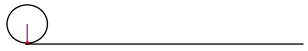Motion of a wheel rolling without slip on a stationary surface

It is surprisingly difficult to visualize the motion of a wheel.  The figure above might help: it shows the trajectory of one point on the circumference of the wheel.  The point traces quite a complicated path.   The important thing to notice is:

If a wheel rolls without slip on a stationary surface, the point touching the surface is stationary

Each point is only in contact with the ground for an instant, and while it touches the ground it has a large vertical acceleration, but it is instantaneously stationary.  We know this from the list of constraints in Sect 6.3.1, of course, but it’s still not an easy thing to visualize.

More generally, the ground need not necessarily be stationary (or the wheel could touch another surface).  In this case we know that the contacting points on two bodies in rolling contact have equal velocity at the contact.

Angular velocity-linear velocity formula: With this insight, we can use the rigid body formulas to calculate the instantaneous velocity vector for any point on the wheel.  Assume that

• The wheel rolls with angular velocity ω = ωzk counterclockwise rotation is positive.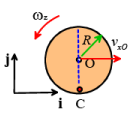• The center of the wheel moves with velocity vO = vxO i The rolling wheel formula gives vxO = −ωzR

To see this, you can simply use the rigid body formula to go from the contact point (which is stationary) to O

vO −vC= ω × (rO − rC ) ⇒ vOzk × (Rj) = −ωzRi

More generally, we can calculate the velocity of any point on the wheel we might be interested in.  In fact, we can just write down the velocity of any point in the wheel by noticing that instantaneously all points are in circular motion about the contact point (just imagine the disk is rotating about C).  See if you can show all the following:

• vA = −ωzR(i+j)
• vD = −ωz2Ri
• vB = −ωzR(i−j)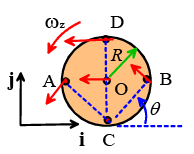Notice that the direction of the velocity at each point is always perpendicular to the line connecting to the point to C.

Angular acceleration-linear acceleration formula: Assume that

• The wheel rolls with angular acceleration α = α zk counterclockwise rotation is positive.
• The center of the wheel moves with acceleration aO = axOi The rolling wheel formula gives

axO = −αzR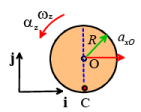You can derive this formula in two different ways:

1. Differentiate the velocity formula vxO = −ω zR with respect to time
2. Use the rigid body formula:

(aO - ac) = α x (rO - rC ) - ω2z(rO - rC)

⇒aO = aCzRi -ω2zRj

We know that the i component of acceleration at point C has to be the same as the i component of acceleration of the ground (i.e. zero).  (The j components don’t have to be equal).   We also know that O has no j acceleration, because it remains at the same height above the ground.   Therefore

axOi= ayCj -azRi -ωz2Rj

⇒ αxO = -αzR αyC = ω2zR

We can calculate the acceleration of any other point on the disk using the rigid body formula.

Example: The block AB has horizontal acceleration α and horizontal speed v.   Calculate the angular velocity and angular acceleration of the rollers. Then, calculate the linear velocity and acceleration of O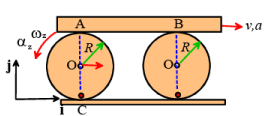To solve problems like this we use two ideas: (1) the formulas relating velocity and accelerations of points on the disk; and (2) the tangential velocity and acceleration of contacting points are equal.

Here, we know the tangential velocity at C is zero; the tangential velocity at A is vi . We can use the wheel formulas

vxA = −2ωz R ⇒ ωz=−v /(2R)

Similarly, the tangential acceleration at A isαi . The rolling wheel formula gives

axA = −2αz R ⇒αz= −α / (2R)

To find the velocity and acceleration at O, we can use

vxO = -ωzR = v/2

αxo = -αzR =α / 2

Offer running on EduRev: Apply code STAYHOME200 to get INR 200 off on our premium plan EduRev Infinity!

## Introduction to Dynamics and Vibrations- Notes, Videos, MCQs

20 videos|53 docs

,

,

,

,

,

,

,

,

,

,

,

,

,

,

,

,

,

,

,

,

,

;